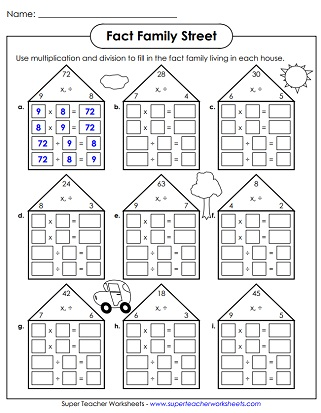# Fact Families / Number Families (Multiply & Divide)

Enjoy these printable fact family and number bond worksheets to show the relationship between multiplication and division.## Fact Family Flashcards

Here's a complete set of triangular multiplication and division fact family flashcards.

## Fact Family Triangles

Another page of multiplication-division fact family triangles.
Write the correct set of fact family problems in each house.
Another worksheet with fact family houses to complete.

## Fact Family Boxes

Use multiplication or division to figure out the missing number in each factor-factor-product box.  Includes facts for 0 - 9.
Another worksheet with factor-factor-product boxes for students to solve.  Includes facts for 0 - 12.

## Fact Family Circles & Number Bonds

Complete these multiplication and division number families.  Includes basic facts for up to 9.
Complete the multiplication number bonds. Then write a fact in the space provided.
Complete these multiplication and division number families.  Includes facts for 0 - 12.
Students fill in the missing numbers for each number bond. Then write the multiplication fact shown by each model.

## Multiplication Arrays

Multiplication Worksheets

Basic multiplication printable worksheets

Division Worksheets

Basic division printable worksheets

Number bonds and fact families for addition and subtraction facts

## Worksheet ImagesMy Account
Site Information